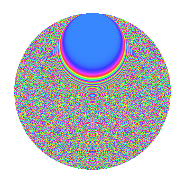# Properties

 Label 1575.2.ekLevel 1575 Weight 2 Character orbit ek Rep. character $$\chi_{1575}(2,\cdot)$$ Character field $$\Q(\zeta_{60})$$ Dimension 3776 Sturm bound 480

# Related objects

## Defining parameters

 Level: $$N$$ = $$1575 = 3^{2} \cdot 5^{2} \cdot 7$$ Weight: $$k$$ = $$2$$ Character orbit: $$[\chi]$$ = 1575.ek (of order $$60$$ and degree $$16$$) Character conductor: $$\operatorname{cond}(\chi)$$ = $$1575$$ Character field: $$\Q(\zeta_{60})$$ Sturm bound: $$480$$

## Dimensions

The following table gives the dimensions of various subspaces of $$M_{2}(1575, [\chi])$$.

Total New Old
Modular forms 3904 3904 0
Cusp forms 3776 3776 0
Eisenstein series 128 128 0

## Trace form

 $$3776q - 24q^{2} - 8q^{3} + 10q^{4} - 24q^{6} - 8q^{7} - 10q^{9} + O(q^{10})$$ $$3776q - 24q^{2} - 8q^{3} + 10q^{4} - 24q^{6} - 8q^{7} - 10q^{9} - 16q^{10} + 12q^{12} - 16q^{13} - 30q^{14} - 26q^{15} - 442q^{16} + 18q^{17} - 20q^{19} - 48q^{20} - 12q^{21} - 24q^{22} - 16q^{25} - 8q^{27} - 36q^{28} - 60q^{29} + 10q^{30} + 6q^{31} + 60q^{32} - 42q^{33} - 20q^{34} - 40q^{36} - 16q^{37} - 50q^{39} + 16q^{40} - 36q^{41} - 34q^{42} - 16q^{43} - 102q^{45} - 12q^{46} - 24q^{47} - 98q^{48} - 96q^{50} - 16q^{51} - 48q^{52} - 10q^{54} - 84q^{55} - 18q^{56} + 8q^{57} - 8q^{58} - 30q^{59} - 36q^{60} + 6q^{61} - 134q^{63} - 80q^{64} + 48q^{65} - 30q^{66} + 8q^{67} - 110q^{69} + 32q^{70} + 36q^{72} - 16q^{73} + 96q^{75} - 16q^{76} - 72q^{77} - 154q^{78} + 10q^{79} - 36q^{80} - 6q^{81} - 12q^{82} - 48q^{83} - 260q^{84} - 16q^{85} + 18q^{87} - 32q^{88} - 150q^{89} - 76q^{90} - 24q^{91} - 132q^{92} - 14q^{93} + 10q^{94} + 36q^{95} - 102q^{96} - 16q^{97} + 48q^{98} + O(q^{100})$$

## Decomposition of $$S_{2}^{\mathrm{new}}(1575, [\chi])$$ into newform subspaces

The newforms in this space have not yet been added to the LMFDB.

## Hecke Characteristic Polynomials

There are no characteristic polynomials of Hecke operators in the database